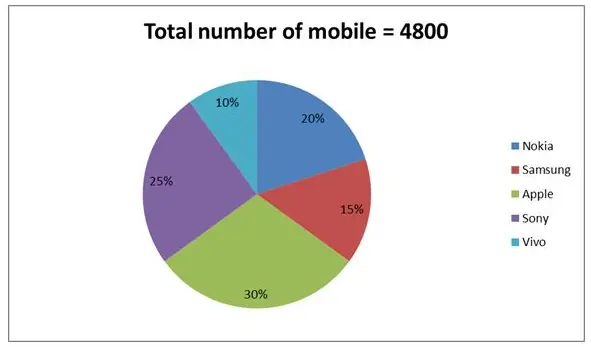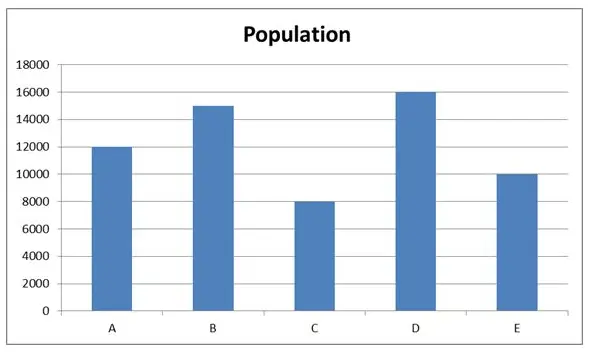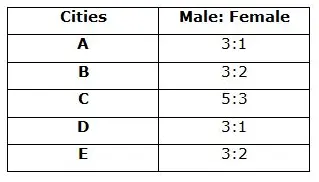# RRB PO Prelims Quantitative Aptitude (Day-09)

Dear Aspirants, Our IBPS Guide team is providing new series of Quantitative Aptitude Questions for RRB PO 2020 Prelims so the aspirants can practice it on a daily basis. These questions are framed by our skilled experts after understanding your needs thoroughly. Aspirants can practice these new series questions daily to familiarize with the exact exam pattern and make your preparation effective.

Start Quiz

IBPS RRB PO Prelims

Data Interpretation

Direction (1-4): Study the following information carefully and answer the questions given below.

The given pie graph shows the number of mobiles in five different companies- Nokia, Samsung, Apple, Sony and Vivo.1) If 70% of the Nokia mobile is sold, find the number of unsold Nokia mobile?

A) 218

B) 267

C) 276

D) 288

E) None of these

2) The number of Samsung mobile is what percent of the number of Sony mobile?

A) 40%

B) 50%

C) 60%

D) 70%

E) None of these

3) What is the average number of Apple, Sony and Vivo mobiles together?

A) 1040

B) 1103

C) 1085

D) 1125

E) None of these

4) What is the ratio of the number of Nokia and Vivo mobile together to the number of apple mobile?

A) 2:1

B) 3:2

C) 4:3

D) 5:4

E) None of these

Data Interpretation

Direction (5-10): Study the following information carefully and answer the questions given below.

The given bar graph shows the number of population in five different cities – A, B, C, D and E in 2018The given table shows the number of male to female population in five different cities.5) What is the total number of female population in City D and E together?

A) 4000

B) 6000

C) 8000

D) 10000

E) None of these

6) What is the average number of male population in all the cities together?

A) 7600

B) 7900

C) 8200

D) 8500

E) None of these

7) What is the difference between the number of female population in B and E together and the number of female population in A and C together?

A) 5000

B) 4000

C) 3000

D) 2000

E) None of these

8) If the ratio of the number of population in below poverty to above poverty in city B is 13: 17, then what is the difference between the number of male population from A and the number of above poverty population in B?

A) 300

B) 400

C) 500

D) 200

E) None of these

9) In 2019 the number of female population in D is increased by 40% compared to previous year and the number of male population in D is decreased by 20% compared to previous year. What is the total number of population in D in 2019?

A) 15200

B) 15600

C) 14900

D) 14960

E) None of these

10) What is the ratio of the number of male population in C and E together to the average number of female population from A, B, D and E together?

A) 38:11

B) 39:16

C) 40:13

D) 44:17

E) None of these

Directions (1-4) :

Number of Nokia mobile = 20/100 * 4800 = 960

Number of unsold Nokia mobile = 960 * 30/100 = 288

Required ratio = 15/25 * 100 = 60%

Apple = 30/100 * 4800 = 1440

Sony = 25/100 * 4800 = 1200

Vivo = 10/100 * 4800 = 480

Average = (1440 + 1200 + 480)/3 = 1040

Required ratio = (20 + 10):30

= 1:1

Direction (5-10):Required total = 4000 + 4000 = 8000

Required Average = (9000 + 9000 + 5000 + 12000 + 6000)/5 = 8200

B and E female population = 6000 + 4000 = 10000

A and C female population = 3000 + 3000 = 6000

Difference = 10000 – 6000 = 4000

Number of above poverty population in B = 17/30 * 15000 = 8500

Number of male population in A = 9000

Difference = 9000 – 8500 = 500

Number of female population in D in 2019 = 4000 * 140/100 = 5600

Number of male population in D in 2019 = 12000 * 80/100 = 9600

Total population in D in 2019 = 5600 + 9600 = 15200

Required ratio = (5000 + 6000):(3000 + 6000 + 4000 + 4000)/4

= 11000:4250

= 220:85

= 44:17

 Check Here to View RRB PO Prelims 2020 Quantitative Aptitude Questions Day – 08 Day – 07 Day – 06 Click Here for RRB 2020 Officer Scale 1 – Detailed Exam Notification